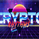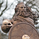5989 views
The slow relative strength index (SRSI) indicator created by Vitali Apirine is a momentum price oscillator similar to RSI in its application and interpretation. Oscillating between 0 and 100, it generates both OB/OS signals and midline (50) cross over signals and divergences.

As author suggests, bullish/bearish divergences generated by SRSI are not as effective during strong trends. To avoid fading an established trend, the system is used in conjunction with a trend confirmation tool like ADX indicator.

You can configure the OB/OS levels, default are 70/30.

The slow relative strength index , TASC 2015-07

List of my public indicators: http://bit.ly/1LQaPK8
List of my app-store indicators: http://blog.tradingview.com/?p=970

```//
// @author LazyBear
//
// List of my public indicators: http://bit.ly/1LQaPK8
// List of my app-store indicators: http://blog.tradingview.com/?p=970
//
study("Apirine Slow RSI [LazyBear]", shorttitle="ASRSI_LB", overlay=false, precision=3)
periods = input( 6, title="Smoothing", minval=1, maxval=100 )
smooth =  input( 14, title="RSI Length", minval=1, maxval=100 )
price = input(close, title="Source")
calc_wima(src, length) =>
MA_s=(src + nz(MA_s * (length-1)))/length
MA_s

r1 = ema( price, periods )
r2 = iff( price > r1, price - r1, 0 )
r3 = iff( price < r1, r1 - price, 0 )
r4 = calc_wima( r2, smooth )
r5 = calc_wima( r3, smooth )
rr = iff( r5 == 0, 100, 100 - ( 100 / ( 1 + ( r4 / r5 ) ) ) )
obl=input(70, title="OB Level")
ovl=input(30, title="OS Level")
obl1=hline(obl, title="OB", color=gray), osl1=hline(ovl, title="OS", color=gray)
fill(obl1,osl1, color=gray, transp=80, title="RegionFill")
hline(50, title="MidLine", color=gray)
plot( rr, title="SlowRSI", color=red, linewidth=2 )
```
List of my free indicators: http://bit.ly/1LQaPK8
List of my indicators at Appstore: http://blog.tradingview.com/?p=970Thanks for this LB. I used your code to make a candlestick version with volume weighting and HA candle options. I hope you dont mind (i did give you credit of course).Looks good LB. Thanks for sharing it and all the other indicators that you provide to us. By the way, I have looked for a Time Series Forecast indicator in the scripts and have not found it. Do you know if it exists? Thanks again.